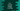# 3 different C++ program to convert integer to string## C++ program to convert integer to string:

In this post, we will learn how to convert a integer to string in C++. We have three different ways to convert an integer to string in C++.

Let’s check them one by one:

## Method 1: By using the stringstream class:

stringstream is used for string stream operations. It uses << and >> operators to read and write data from the stream.

So, we can use stringstream to convert an integer to string.

We can create one stringstream object and read the integer to it as stream. Then, we can write the data to a string object.

Below program uses stringstream to convert an integer to string:

``````#include <iostream>
#include <sstream>

using namespace std;

int main()
{
int givenNumber = 111;

string convertedString;

stringstream stream;

stream << givenNumber;
stream >> convertedString;

cout << "Given number: " << givenNumber << ", converted string: " << convertedString << endl;

return 0;
}``````
• sstream is needed to use stringstream
• givenNumber is the given number.
• convertedString is the string converted from the number givenNumber.
• We are using a stringstream to converted the integer to string.

If you run this program, it will print the below output:

``Given number: 111, converted string: 111``

As you can see here, the integer is converted to a string.

## Method 2: By using to_string():

to_string method is used for converting any numerical value to a string. This method can take any numerical value as the parameter.

It is defined as like below:

``````string to_string (unsigned val);
string to_string (unsigned long val);
string to_string (unsigned long long val);

string to_string (int val);
string to_string (long val);
string to_string (long long val);
string to_string (long double val);

string to_string (float val);
string to_string (double val);``````

As you can see, it can take different types of numerical argument and it converts it to its string equivalent and returns that.

Let’s try to convert an integer to string by using the to_string method:

``````#include <iostream>
#include<string>

using namespace std;

int main()
{
int givenNumber = 111;
string convertedString = to_string(givenNumber);

cout << "Given number: " << givenNumber << ", converted string: " << convertedString << endl;

return 0;
}``````

It will print:

``Given number: 111, converted string: 111``

## Method 3: By using boost/lexical_cast.hpp:

By using boost C++ library’s function lexical_cast, we can convert an integer to a string value. lexical_cast does conversion in between other data-types as well.

The below program uses lexical_cast to convert an integer to string:

``````#include <iostream>
#include <boost/lexical_cast.hpp>

using namespace std;

int main()
{
int givenNumber = 111;
string convertedString = boost::lexical_cast<string>(givenNumber);

cout << "Given number: " << givenNumber << ", converted string: " << convertedString << endl;

return 0;
}``````

It will print similar output.